# AP Board 9th Class Maths Solutions Chapter 13 Geometrical Constructions Ex 13.2

AP State Syllabus AP Board 9th Class Maths Solutions Chapter 13 Geometrical Constructions Ex 13.2 Textbook Questions and Answers.

## AP State Syllabus 9th Class Maths Solutions 13th Lesson Geometrical Constructions Exercise 13.2Question 1.
Construct AABC in which BC = 7 cm,∠B = 75° and AB + AC =12 cm.
Solution:
A.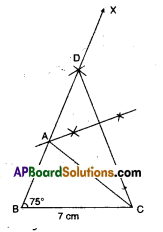Steps:

• Draw a line segment BC = 7 cm.
• Erect ∠B = 75°
• MarkapointDon $$\overrightarrow{\mathrm{BX}}$$ suchthat BD = AB + AC.
• Join D, C and draw the perpendicular bisector of $$\overline{\mathrm{CD}}$$ meeting BD at A.
• Join A to C to form the ΔABC.Question 2.
Construct ΔPQR in which QR = 8 cm, ∠B = 60° and AB – AC = 3.5 cm.
Read ∠Q = 60°and PQ – PR = 3.5 cm
Solution:
A.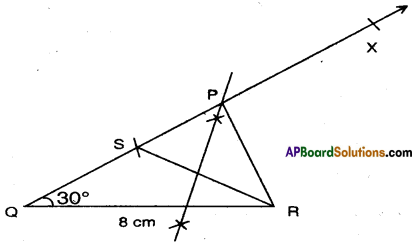Steps: I

• Draw QR = 8 cm.
• Construct ∠RQX = 300 at Q.
• Mark a point S on $$\overrightarrow{\mathrm{QX}}$$ such that QS = PQ  –  PR = 3.5 cm.
• Join S, R and draw the perpendicular bisector to $$\overline{\mathrm{QR}}$$ meeting $$\overrightarrow{\mathrm{QX}}$$ at P.
• Join P, R to form the ΔPQR.Question 3.
Construct ΔXYZ in which ∠Y = 30 °; ∠Z = 60 ° and XY + YZ + ZX = 10 cm.
Solution:
A.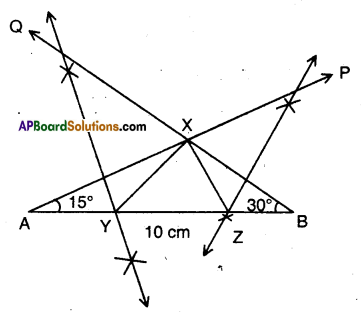Steps:

• Draw a line segment AB = XY + YZ + ZX = 10 cm.
• Construct ∠BAP = $$\frac { 1 }{ 2 }$$ ∠Y at A and ∠ABQ = $$\frac { 1 }{ 2 }$$ ∠Z at B meeting at X.
• Draw the perpendicular bisectors to XA and XB meeting $$\overline{\mathrm{AB}}$$ at Y and Z respectively.
• Join X to Y and Z to form the ΔXYZ.Question 4.
Construct a right triangle whose base is 7.5 cm and sum of its hypotenuse and otherside is 15 cm.
Solution:
A.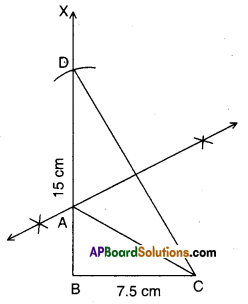Steps:

• Draw BC = 7.5 cm.
• Construct ∠CBX = 90°
• Mark a point D on $$\overrightarrow{\mathrm{BX}}$$ such that BD = 15 cm.
• Join C, D. ‘
• Draw the perpendicular bisectors of $$\overline{\mathrm{CD}}$$ meeting BD at A.
• Join A, C to form the ΔABC.Question 5.
Construct a segment of a circle on a chord of length 5 cm containing the following angles i) 90° ii) 45° iii) 120°
Solution:
i) 90°
A.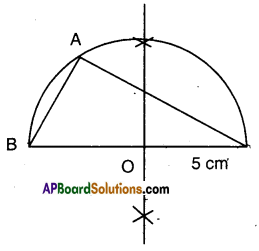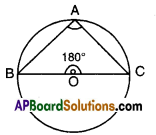Steps:

• Draw a rough sketch of ∠BAC = 90° and ∠BOC = 180°.
• Draw a line segment BC = 5 cm.
• Draw the perpendicular bisector of BC meeting $$\overline{\mathrm{BC}}$$ at O
• Draw an arc of radius OB or OC with centre O.
• Mark any point A on the arc and join it with B and C.
• ∠BAC = 90°

ii) 45°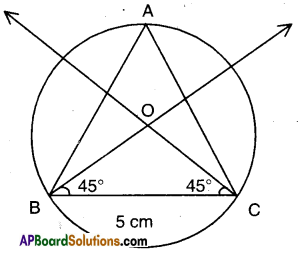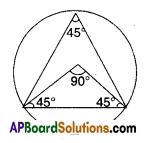Steps:

• Draw a line segment BC = 5 cm.
• Construct ABOC such that BC = 5 cm, ∠B = 45° = ∠C.
• Draw a circle segment of radius OB or OC with centre ’O’.
• Mark any point A on the segment and join it with B and C.
• ∠BAC = 45°iii) 120°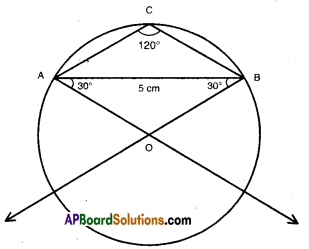Steps:

• Draw a line segment AB = 5 cm. ,
• Construct ΔAOB in which ∠A = 30°; ∠B = 30°; AB = 5 cm.
• With ‘O’ as centre draw a circle segment.
• On the opposite side make any point C and join it with B and C.
• ∠ACB = 120°